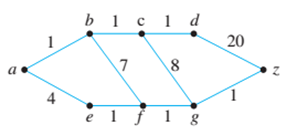Chapter 10.6, Problem 14ES### Discrete Mathematics With Applicat...

5th Edition
EPP + 1 other
ISBN: 9781337694193

#### Solutions

Chapter
Section### Discrete Mathematics With Applicat...

5th Edition
EPP + 1 other
ISBN: 9781337694193
Textbook Problem
8 views

# Use Dijkstra’s algorithm to find the shortest path from a to z for each of the graphs in 13—16. In each case make tables similar to Table 10.6.1 to show the action of the algorithm. 14.To determine

The shortest path for the given problem using Dijkstra’s algorithm.

Explanation

Given information:

A graph G is given as,

Calculation:

Now, calculate the shortest path from a to z using Dijkstra’s algorithm.

 Iteration V(T) E(T) F 012345678 {a}{a}{a,b}{a,b,c}{a,b,c,d}{a,b,c,d,e}{a,b,c,d,e,f}{a,b,c,d,e,f,g}{a,b,c,d,e,f,g,z} ∅∅{{a,b}}{{a,b},{b,c}}{{a,b},{b,c},{c,d}}{{a,b},{b,c},{c,d},{a,e}}{{a,b},{b,c},{c,d},{a,e},{e,f}}{{a,b},{b,c},{c,d},{a,e},{e,f},{f,g}}{{a,b},{b,c},{c,d},{a,e},{e,f},{f,g},{g,z}} {a}

### Still sussing out bartleby?

Check out a sample textbook solution.

See a sample solution

#### The Solution to Your Study Problems

Bartleby provides explanations to thousands of textbook problems written by our experts, many with advanced degrees!

Get Started